2012-03-18 16:03:49 leo_wonty 阅读数 8325
• ###### SAP快速入门

帮助学员，快速形成对ERP和SAP的基本认识，达到快速入门的目标。 完成课程学习后，将理解以下基本概念 -  * MRP，ERP，SAP NetWeaver，组件，模块， * 最佳实践，三层架构，三系统模型，组织要素，主数据，业务数据， * ECC, S/4HANA，Fiori 等 并学会以下基本操作 -  * 登录SAP系统，系统导航，收藏夹，系统操作的快捷方式， * 运行一个事务代码，选择屏幕，F1/F4帮助，输出控制等

809 人正在学习 去看看 国峰

ECC（Elliptic Curves Cryptography）加密算法是一种公钥加密算法，与主流的RSA算法相比，ECC算法可以使用较短的密钥达到相同的安全程度。近年来，人们对ECC的认识已经不再处于研究阶段，开始逐步进入实际应用，如国家密码管理局颁布的SM2算法就是基于ECC算法的。下面我们来认识一下ECC的工作原理。

### 椭圆曲线

#### 定义

在引入椭圆曲线之前，不得不提到一种新的坐标系-------射影平面坐标系，它是对笛卡尔直角坐标系的扩展，增加了无穷远点的概念。在此坐标系下，两条平行的直线是有交点的，而交点就是无穷远点。两者的变换关系为：

y2+a1xy+a3y = x3+a2x2+a4x+a6

#### 加法法则• 此处+不是简单的实数相加，是抽象出来的
• O∞+P=P，O∞为零元
• 曲线上三个点A,B,C处于一条直线上，则A+B+C=O∞

下面，我们利用P、Q点的坐标(x1,y1)，(x2,y2)，求出R=P+Q的坐标(x4,y4)。

P,Q,R'共线，设为y=kx+b，

x4=k2+ka1-a2-x1-x2
y4=k(x1-x4)-y1-a1x4-a3;

### 密码学中的椭圆曲线

#### 定义

1. Fp中只有p个元素，p为素数
2. Fp中，a+b≡c (mod p)，a×b≡c (mod p)，a/b≡c (mod p)
3. 4a3+27b2≠0　(mod p)  a，b是小于p的非负整数
4. x，y属于0到p-1间的证书，曲线标记为Ep（a，b）

#### 椭圆曲线难题

K=kG，其中K,G为Ep（a,b）上的点，k为小于n的整数，n是点G的阶，给定k和G，计算K容易，但是给定K和G，求k就很难了！

#### 加密过程

1. A选定一条椭圆曲线Ep（a,b），并取曲线上一点作为基点G
2. A选择一个私钥k，并生成公钥K=kG
3. A将Ep（a,b）和k，G发送给B
4. B收到后将明文编码到Ep（a,b）上一点M，并产生一个随机数r
5. B计算点C1=M+rK，C2=rG
6. B将C1，C2传给A
7. A计算C1-kC2=M+rkG-krG=M
8. A对M解码得到明文

#### 签名验签流程

1. A选定一条椭圆曲线Ep（a，b），并取曲线上一点作为基点G
2. A选择一个私钥k，并生成公钥K=kG
3. A产生一个随机数r，计算R(x,y)=rG
4. A计算Hash=SHA(M)，M‘=M(modp)
5. A计算S=（Hash+M'k）/r(modp)
6. B获得S和M'，Ep(a,b)，K，R(x,y)
7. B计算Hash=SHA(M)，M'=M(modp)
8. B计算R'=（Hash*G+M'*K）/S=(Hash*G+M'*kG)*r/(Hash+M'k)=rG=R（x,y），若R'=R，则验签成功。

ecc算法 swift 相关内容

2017-03-01 23:26:34 scuyxi 阅读数 15499
• ###### SAP快速入门

帮助学员，快速形成对ERP和SAP的基本认识，达到快速入门的目标。 完成课程学习后，将理解以下基本概念 -  * MRP，ERP，SAP NetWeaver，组件，模块， * 最佳实践，三层架构，三系统模型，组织要素，主数据，业务数据， * ECC, S/4HANA，Fiori 等 并学会以下基本操作 -  * 登录SAP系统，系统导航，收藏夹，系统操作的快捷方式， * 运行一个事务代码，选择屏幕，F1/F4帮助，输出控制等

809 人正在学习 去看看 国峰

OpenSSL实现的ECC 算法，包括三部分： ECC 算法(crypto/ec)、椭圆曲线数字签名算法 ECDSA (crypto/ecdsa)以及椭圆曲线密钥交换算法 ECDH(crypto/dh)。

## 密钥数据结构

``````struct ec_key_st {
const EC_KEY_METHOD *meth;
ENGINE *engine;
int version;
EC_GROUP *group; //密钥参数
EC_POINT *pub_key;
BIGNUM *priv_key;
unsigned int enc_flag;
point_conversion_form_t conv_form;
int references;
int flags;
CRYPTO_EX_DATA ex_data;
CRYPTO_RWLOCK *lock;
};``````

## 签名值数据结构

``````struct ECDSA_SIG_st {
BIGNUM *r;
BIGNUM *s;
};``````

## 签名与验签

crypto/ec/ ecdsa_sign.c 实现了签名算法，
crypto/ec/ ecdsa_vrf.c 实现了验签

## 密钥交换

crypto/ec/ec dh_ossl.c 实现了密钥交换算法。

## 主要函数

1) EC_get_builtin_curves

``````size_t EC_get_builtin_curves(EC_builtin_curve *r, size_t nitems)
{
size_t i, min;

if (r == NULL || nitems == 0)
return curve_list_length;

min = nitems < curve_list_length ? nitems : curve_list_length;

for (i = 0; i < min; i++) {
r[i].nid = curve_list[i].nid;
r[i].comment = curve_list[i].comment;
}

return curve_list_length;
}``````

2) EC_GROUP_new_by_curve_name

``````EC_GROUP *EC_GROUP_new_by_curve_name(int nid)
{
size_t i;
EC_GROUP *ret = NULL;

if (nid <= 0)
return NULL;

for (i = 0; i < curve_list_length; i++)
if (curve_list[i].nid == nid) {
ret = ec_group_new_from_data(curve_list[i]);
break;
}

if (ret == NULL) {
ECerr(EC_F_EC_GROUP_NEW_BY_CURVE_NAME, EC_R_UNKNOWN_GROUP);
return NULL;
}

EC_GROUP_set_curve_name(ret, nid);

return ret;
}``````

3) int EC_KEY_generate_key

``````int EC_KEY_generate_key(EC_KEY *eckey)
{
if (eckey == NULL || eckey->group == NULL) {
ECerr(EC_F_EC_KEY_GENERATE_KEY, ERR_R_PASSED_NULL_PARAMETER);
return 0;
}
if (eckey->meth->keygen != NULL)
return eckey->meth->keygen(eckey);
ECerr(EC_F_EC_KEY_GENERATE_KEY, EC_R_OPERATION_NOT_SUPPORTED);
return 0;
}``````

4) int EC_KEY_check_key

``````int EC_KEY_check_key(const EC_KEY *eckey)
{
if (eckey == NULL || eckey->group == NULL || eckey->pub_key == NULL) {
ECerr(EC_F_EC_KEY_CHECK_KEY, ERR_R_PASSED_NULL_PARAMETER);
return 0;
}

if (eckey->group->meth->keycheck == NULL) {
ECerr(EC_F_EC_KEY_CHECK_KEY, ERR_R_SHOULD_NOT_HAVE_BEEN_CALLED);
return 0;
}

return eckey->group->meth->keycheck(eckey);
}``````

5) int ECDSA_size

``````int ECDSA_size(const EC_KEY *r)
{
int ret, i;
ASN1_INTEGER bs;
unsigned char buf;
const EC_GROUP *group;

if (r == NULL)
return 0;
group = EC_KEY_get0_group(r);
if (group == NULL)
return 0;

i = EC_GROUP_order_bits(group);
if (i == 0)
return 0;
bs.length = (i + 7) / 8;
bs.data = buf;
bs.type = V_ASN1_INTEGER;
/* If the top bit is set the asn1 encoding is 1 larger. */
buf = 0xff;

i = i2d_ASN1_INTEGER(&bs, NULL);
i += i;                     /* r and s */
ret = ASN1_object_size(1, i, V_ASN1_SEQUENCE);
return (ret);
}``````

6) ECDSA_sign

``````int ECDSA_sign(int type, const unsigned char *dgst, int dlen, unsigned char
*sig, unsigned int *siglen, EC_KEY *eckey)
{
return ECDSA_sign_ex(type, dgst, dlen, sig, siglen, NULL, NULL, eckey);
}

int ECDSA_sign_ex(int type, const unsigned char *dgst, int dlen,
unsigned char *sig, unsigned int *siglen, const BIGNUM *kinv,
const BIGNUM *r, EC_KEY *eckey)
{
if (eckey->meth->sign != NULL)
return eckey->meth->sign(type, dgst, dlen, sig, siglen, kinv, r, eckey);
ECerr(EC_F_ECDSA_SIGN_EX, EC_R_OPERATION_NOT_SUPPORTED);
return 0;
}``````

7) ECDSA_verify

``````/*-
* returns
*      1: correct signature
*      0: incorrect signature
*     -1: error
*/
int ECDSA_verify(int type, const unsigned char *dgst, int dgst_len,
const unsigned char *sigbuf, int sig_len, EC_KEY *eckey)
{
if (eckey->meth->verify != NULL)
return eckey->meth->verify(type, dgst, dgst_len, sigbuf, sig_len,
eckey);
ECerr(EC_F_ECDSA_VERIFY, EC_R_OPERATION_NOT_SUPPORTED);
return 0;
}``````

8) EC_KEY_get0_public_key

``````const EC_POINT *EC_KEY_get0_public_key(const EC_KEY *key)
{
return key->pub_key;
}

int EC_KEY_set_public_key(EC_KEY *key, const EC_POINT *pub_key)
{
if (key->meth->set_public != NULL
&& key->meth->set_public(key, pub_key) == 0)
return 0;
EC_POINT_free(key->pub_key);
key->pub_key = EC_POINT_dup(pub_key, key->group);
return (key->pub_key == NULL) ? 0 : 1;
}``````

9)EC_KEY_get0_private_key

``````const BIGNUM *EC_KEY_get0_private_key(const EC_KEY *key)
{
return key->priv_key;
}

int EC_KEY_set_private_key(EC_KEY *key, const BIGNUM *priv_key)
{
if (key->group == NULL || key->group->meth == NULL)
return 0;
if (key->group->meth->set_private != NULL
&& key->group->meth->set_private(key, priv_key) == 0)
return 0;
if (key->meth->set_private != NULL
&& key->meth->set_private(key, priv_key) == 0)
return 0;
BN_clear_free(key->priv_key);
key->priv_key = BN_dup(priv_key);
return (key->priv_key == NULL) ? 0 : 1;
}``````
1. ECDH_compute_key
生成共享密钥
``````int ECDH_compute_key(void *out, size_t outlen, const EC_POINT *pub_key,
const EC_KEY *eckey,
void *(*KDF) (const void *in, size_t inlen, void *out,
size_t *outlen))
{
unsigned char *sec = NULL;
size_t seclen;
if (eckey->meth->compute_key == NULL) {
ECerr(EC_F_ECDH_COMPUTE_KEY, EC_R_OPERATION_NOT_SUPPORTED);
return 0;
}
if (outlen > INT_MAX) {
ECerr(EC_F_ECDH_COMPUTE_KEY, EC_R_INVALID_OUTPUT_LENGTH);
return 0;
}
if (!eckey->meth->compute_key(&sec, &seclen, pub_key, eckey))
return 0;
if (KDF != NULL) {
KDF(sec, seclen, out, &outlen);
} else {
if (outlen > seclen)
outlen = seclen;
memcpy(out, sec, outlen);
}
OPENSSL_clear_free(sec, seclen);
return outlen;
}``````

## 编程示例

``````
#include <string.h>
#include <stdio.h>
#include <openssl/ec.h>
#include <openssl/ecdh.h>
#include <openssl/ecdsa.h>
#include <openssl/objects.h>
#include <openssl/err.h>
int main()
{
EC_KEY *key1,*key2;
const EC_POINT *pubkey1,*pubkey2;
EC_GROUP *group1,*group2;
unsigned int ret,nid,size,i,sig_len;
unsigned char *signature,digest;
BIO *berr;
EC_builtin_curve *curves;
int crv_len;
char shareKey1,shareKey2;
int len1,len2;
/* 构造 EC_KEY 数据结构 */
key1=EC_KEY_new();
if(key1==NULL)
{
printf("EC_KEY_new err!\n");
return -1;
}
key2=EC_KEY_new();
if(key2==NULL)
{
printf("EC_KEY_new err!\n");
return -1;
}
/* 获取实现的椭圆曲线个数 */
crv_len = EC_get_builtin_curves(NULL, 0);
curves = (EC_builtin_curve *)malloc(sizeof(EC_builtin_curve) * crv_len);
/* 获取椭圆曲线列表 */
EC_get_builtin_curves(curves, crv_len);
/*
nid=curves.nid;会有错误，原因是密钥太短
*/
/* 选取一种椭圆曲线 */
nid=curves.nid;
/* 根据选择的椭圆曲线生成密钥参数 group */
group1=EC_GROUP_new_by_curve_name(nid);
if(group1==NULL)
{
printf("EC_GROUP_new_by_curve_name err!\n");
return -1;
}
group2=EC_GROUP_new_by_curve_name(nid);
if(group1==NULL)
{
printf("EC_GROUP_new_by_curve_name err!\n");
return -1;
}
/* 设置密钥参数 */
ret=EC_KEY_set_group(key1,group1);
if(ret!=1)
{
printf("EC_KEY_set_group err.\n");
return -1;
}
ret=EC_KEY_set_group(key2,group2);
if(ret!=1)
{
printf("EC_KEY_set_group err.\n");
return -1;
}
/* 生成密钥 */
ret=EC_KEY_generate_key(key1);
if(ret!=1)
{
printf("EC_KEY_generate_key err.\n");
return -1;
}
ret=EC_KEY_generate_key(key2);
if(ret!=1)
{
printf("EC_KEY_generate_key err.\n");
return -1;
}
/* 检查密钥 */
ret=EC_KEY_check_key(key1);
if(ret!=1)
{
printf("check key err.\n");
return -1;
}
/* 获取密钥大小 */
size=ECDSA_size(key1);
printf("size %d \n",size);
for(i=0;i<20;i++)
memset(&digest[i],i+1,1);
signature= (unsigned char*)malloc(size);
berr=BIO_new(BIO_s_file());
//BIO_set_fp(berr,stdout,BIO_NOCLOSE);
/* 签名数据，本例未做摘要，可将 digest 中的数据看作是 sha1 摘要结果 */
ret=ECDSA_sign(0,digest,20,signature,&sig_len,key1);
if(ret!=1)
{
ERR_print_errors(berr);
printf("sign err!\n");
return -1;
}
/* 验证签名 */
ret=ECDSA_verify(0,digest,20,signature,sig_len,key1);
if(ret!=1)
{
ERR_print_errors(berr);
printf("ECDSA_verify err!\n");
return -1;
}
/* 获取对方公钥，不能直接引用 */
pubkey2 = EC_KEY_get0_public_key(key2);
/* 生成一方的共享密钥 */
len1= ECDH_compute_key(shareKey1, 128, pubkey2, key1, NULL);
pubkey1 = EC_KEY_get0_public_key(key1);
/* 生成另一方共享密钥 */
len2= ECDH_compute_key(shareKey2, 128, pubkey1, key2, NULL);
if(len1!=len2)
{
printf("err\n");
}
else
{
ret=memcmp(shareKey1,shareKey2,len1);
if(ret==0)
printf("生成共享密钥成功\n");
else
printf("生成共享密钥失败\n");
}
printf("test ok!\n");
BIO_free(berr);
EC_KEY_free(key1);
EC_KEY_free(key2);
free(signature);
free(curves);
return 0;
}``````ecc算法 swift 相关内容

2010-04-29 18:50:00 dog250 阅读数 5456
• ###### SAP快速入门

帮助学员，快速形成对ERP和SAP的基本认识，达到快速入门的目标。 完成课程学习后，将理解以下基本概念 -  * MRP，ERP，SAP NetWeaver，组件，模块， * 最佳实践，三层架构，三系统模型，组织要素，主数据，业务数据， * ECC, S/4HANA，Fiori 等 并学会以下基本操作 -  * 登录SAP系统，系统导航，收藏夹，系统操作的快捷方式， * 运行一个事务代码，选择屏幕，F1/F4帮助，输出控制等

809 人正在学习 去看看 国峰

但是我们国家总是喜欢搞zg特色，正如我们国家搞双证书体系一样，我们国家的双证书体系为的是方便官方取证，这在别的国家是不存在的，在别的国家，你的私钥是任何机构都无权查看的，可是...公正乎？哀哉！正是因为我们的ecc标准没有公布椭圆曲线参数，于是我们就不能使用openssl了，但是openssl实在太棒了，我无法舍弃它，于是就要想办法将openssl改造成支持国家密码局的ecc标准的框架。要做到这个，首先的想法就是让EC_METHO支持engine，由于国家不公布椭圆曲线标准，那么我们只好调用国家的接口来自己实现。怎么让EC_METHOD支持engine呢？很简单，首先将自己的engine注册进openssl，然后修改获取group的代码，如果需要处理的密钥的oid是我们国家的标准，那么就获取我们实现的engine，之后就用我们的engine的实现的方法来实现曲线操作。
实现了曲线操作，接下来的密钥操作就简单了，密钥操作都是在“曲线”上进行的，只要我们加载自己的engine，那么很容易就可以使得我们自己的engine得到使用，这个就不必多说了，详细情况请看关于engine的介绍。这个工作简单的原因在于ecdh或者ecdsa等本来就是支持engine的。为了实现国密的ecc算法，或者说将国密ecc整合进openssl，我们需要很多的工作要做，我们之所以值得做这些工作原因有二：第一，国家的标准我们必须实现；第二，openssl实在太棒了！

ecc算法 swift 相关内容

2018-11-27 11:48:20 weixin_36793356 阅读数 3184
• ###### SAP快速入门

帮助学员，快速形成对ERP和SAP的基本认识，达到快速入门的目标。 完成课程学习后，将理解以下基本概念 -  * MRP，ERP，SAP NetWeaver，组件，模块， * 最佳实践，三层架构，三系统模型，组织要素，主数据，业务数据， * ECC, S/4HANA，Fiori 等 并学会以下基本操作 -  * 登录SAP系统，系统导航，收藏夹，系统操作的快捷方式， * 运行一个事务代码，选择屏幕，F1/F4帮助，输出控制等

809 人正在学习 去看看 国峰

1.实验内容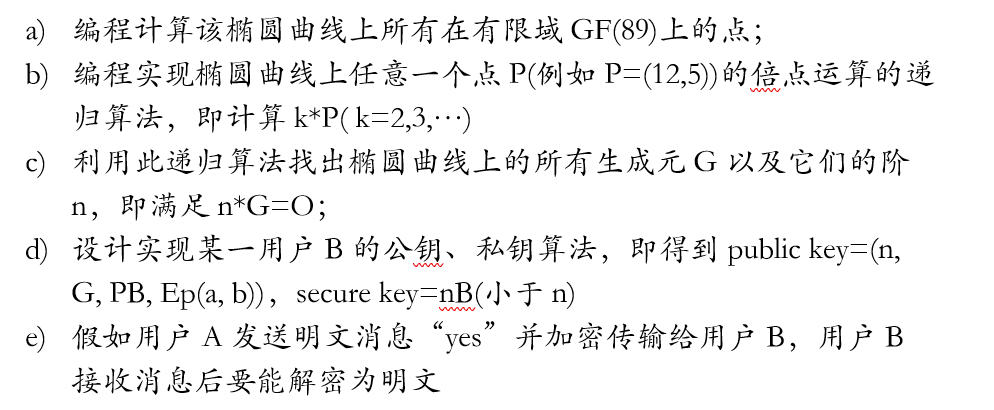2.运行结果：

1.椭圆曲线上的点集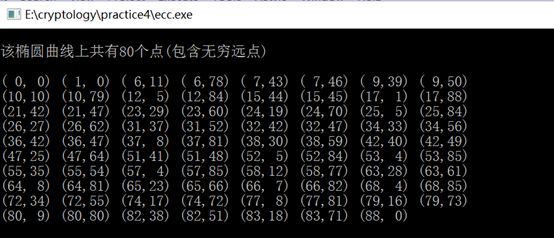2.椭圆曲线生成元以及对应的阶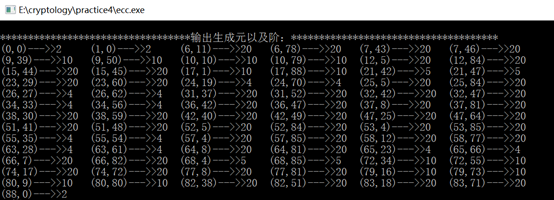3.加解密算法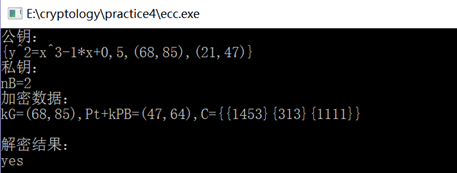``````/*

(1)编程计算该椭圆曲线上所有在有限域GF(89)上的点；
(2)编程实现椭圆曲线上任意一个点P(例如P=(12,5))的倍点运算的递归算法，即计算k*P( k=2,3,…)；（重点！）
(3)利用此递归算法找出椭圆曲线上的所有生成元G以及它们的阶n，即满足n*G=O；
(4)设计实现某一用户B的公钥、私钥算法，即得到public key=(n, G, PB, Ep(a, b))
secure key=nB(小于n)
(5)假如用户A发送明文消息“yes”并加密传输给用户B，用户B接收消息后要能解密为明文。试用ECC密码体制实现此功能。

*/

#include<stdio.h>
#include<stdlib.h>
#include<string.h>
#include<math.h>
#include<time.h>
#define MAX 100

typedef struct point{
int point_x;
int point_y;
}Point;
typedef struct ecc{
struct point p[MAX];
int len;
}ECCPoint;
typedef struct generator{
Point p;
int p_class;
}GENE_SET;

void get_all_points();
int int_sqrt(int s);
Point timesPiont(int k,Point p);
int inverse(int n,int b);
void get_generetor_class();
void encrypt_ecc();
void decrypt_ecc();
int mod_p(int s);
void print();
int isPrime(int n);

char alphabet="abcdefghijklmnopqrstuvwxyz";
int a=-1,b=0,p=89;//椭圆曲线为E89(-1,0)： y2=x3-x (mod 89)
ECCPoint eccPoint;
GENE_SET geneSet[MAX];
int geneLen;
char plain[]="yes";
int m[MAX];
int cipher[MAX];
int nB;//私钥
Point P1,P2,Pt,G,PB;
Point Pm;
int C[MAX];

int main()
{
get_generetor_class();
encrypt_ecc();
decrypt_ecc();
return 0;
}
void encrypt_ecc()
{
int num,i,j;
int gene_class;
int num_t;
int k;
srand(time(NULL));
//明文转换过程
for(i=0;i<strlen(plain);i++)
{
for(j=0;j<26;j++) //for(j=0;j<26;j++)
{
if(plain[i]==alphabet[j])
{
m[i]=j;//将字符串明文换成数字，并存到整型数组m里面
}
}
}
//选择生成元
num=rand()%geneLen;
gene_class=geneSet[num].p_class;
while(isPrime(gene_class)==-1)//不是素数
{
num=rand()%(geneLen-3)+3;
gene_class=geneSet[num].p_class;
}
//printf("gene_class=%d\n",gene_class);
G=geneSet[num].p;
//printf("G:(%d,%d)\n",geneSet[num].p.point_x,geneSet[num].p.point_y);
nB=rand()%(gene_class-1)+1;//选择私钥
PB=timesPiont(nB,G);
printf("\n公钥：\n");
printf("{y^2=x^3%d*x+%d,%d,(%d,%d),(%d,%d)}\n",a,b,gene_class,G.point_x,G.point_y,PB.point_x,PB.point_y);
printf("私钥：\n");
printf("nB=%d\n",nB);
//加密
//
k=rand()%(gene_class-2)+1;
P1=timesPiont(k,G);
//
num_t=rand()%eccPoint.len; //选择映射点
Pt=eccPoint.p[num_t];
//printf("Pt:(%d,%d)\n",Pt.point_x,Pt.point_y);
P2=timesPiont(k,PB);
printf("加密数据：\n");
printf("kG=(%d,%d),Pt+kPB=(%d,%d),C={",P1.point_x,P1.point_y,Pm.point_x,Pm.point_y);
for(i=0;i<strlen(plain);i++)
{
//		num_t=rand()%eccPoint.len; //选择映射点
//		Pt=eccPoint.p[num_t];
C[i]=m[i]*Pt.point_x+Pt.point_y;
printf("{%d}",C[i]);
}
printf("}\n");
}
void decrypt_ecc()
{
Point temp,temp1;
int m,i;
temp=timesPiont(nB,P1);
temp.point_y=0-temp.point_y;
//	printf("(%d,%d)\n",temp.point_x,temp.point_y);
//	printf("(%d,%d)\n",temp1.point_x,temp1.point_y);
printf("\n解密结果：\n");
for(i=0;i<strlen(plain);i++)
{
m=(C[i]-temp1.point_y)/temp1.point_x;
printf("%c",alphabet[m]);//输出密文
}
printf("\n");
}
//判断是否为素数
int isPrime(int n)
{
int i,k;
k = sqrt(n);
for (i = 2; i <= k;i++)
{
if (n%i == 0)
break;
}
if (i <=k){
return -1;
}
else {
return 0;
}
}
void get_generetor_class()
{
int i,j=0;
int count=1;
Point p1,p2;
get_all_points();
//	p1.point_x=p2.point_x=3;
//	p1.point_y=p2.point_y=2;
//	while(1)
//	{
//		printf("(%d,%d)+(%d,%d)---%d\n",p1.point_x,p1.point_y,p2.point_x,p2.point_y,count);
//		count++;
//		if(p2.point_x==-1 && p2.point_y==-1)
//		{
//			break;
//		}
//	}
//	printf("\n\n(%d,%d)---%d\n",p1.point_x,p1.point_y,count);
//
//	do{
//			printf("(%d,%d)+(%d,%d)---%d\n",p1.point_x,p1.point_y,p2.point_x,p2.point_y,count);
//			count++;
//
//	} while(!((p2.point_x==p1.point_x)&&(p2.point_y==p1.point_y)));
//	printf("(%d,%d)+(%d,%d)---%d\n",p1.point_x,p1.point_y,p2.point_x,p2.point_y,count);
//	count ++ ;
//	printf("\n\n(%d,%d)---%d\n",p1.point_x,p1.point_y,count);
printf("\n**********************************输出生成元以及阶：*************************************\n");
for(i=0;i<eccPoint.len;i++)
{
count=1;
p1.point_x=p2.point_x=eccPoint.p[i].point_x;
p1.point_y=p2.point_y=eccPoint.p[i].point_y;
while(1)
{
if(p2.point_x==-1 && p2.point_y==-1)
{
break;
}
count++;
if(p2.point_x==p1.point_x)
{
break;
}
}
count++;
if(count<=eccPoint.len+1)
{
geneSet[j].p.point_x=p1.point_x;
geneSet[j].p.point_y=p1.point_y;
geneSet[j].p_class=count;
printf("(%d,%d)--->>%d\t",geneSet[j].p.point_x,geneSet[j].p.point_y,geneSet[j].p_class);
j++;
if(j % 6 ==0){
printf("\n");
}
}
geneLen=j;
}
}

Point timesPiont(int k,Point p0)
{
if(k==1){
return p0;
}
else if(k==2){
}else{
}
}

//两点的加法运算
{
long t;
int x1=p1.point_x;
int y1=p1.point_y;
int x2=p2.point_x;
int y2=p2.point_y;
int tx,ty;
int x3,y3;
int flag=0;
//求
if((x2==x1)&& (y2==y1) )
{
//相同点相加
if(y1==0)
{
flag=1;
}else{
t=(3*x1*x1+a)*inverse(p,2*y1) % p;
}
//printf("inverse(p,2*y1)=%d\n",inverse(p,2*y1));
}else{
//不同点相加
ty=y2-y1;
tx=x2-x1;
while(ty<0)
{
ty+=p;
}
while(tx<0)
{
tx+=p;
}
if(tx==0 && ty !=0)
{
flag=1;
}else{
t=ty*inverse(p,tx) % p;
}
}
if(flag==1)
{
p2.point_x=-1;
p2.point_y=-1;
}else{
x3=(t*t-x1-x2) % p;
y3=(t*(x1-x3)-y1) % p;
//使结果在有限域GF(P)上
while(x3<0)
{
x3+=p;
}
while(y3<0)
{
y3+=p;
}
p2.point_x=x3;
p2.point_y=y3;
}
return p2;
}
//求b关于n的逆元
int inverse(int n,int b)
{
int q,r,r1=n,r2=b,t,t1=0,t2=1,i=1;
while(r2>0)
{
q=r1/r2;
r=r1%r2;
r1=r2;
r2=r;
t=t1-q*t2;
t1=t2;
t2=t;
}
if(t1>=0)
return t1%n;
else{
while((t1+i*n)<0)
i++;
return t1+i*n;
}
}
void get_all_points()
{
int i=0;
int j=0;
int s,y=0;
int n=0,q=0;
int modsqrt=0;
int flag=0;
if (4 * a * a * a + 27 * b * b != 0)
{
for(i=0;i<=p-1;i++)
{
flag=0;
n=1;
y=0;
s= i * i * i + a * i + b;
while(s<0)
{
s+=p;
}
s=mod_p(s);
modsqrt=int_sqrt(s);
if(modsqrt!=-1)
{
flag=1;
y=modsqrt;
}else{
while(n<=p-1)
{
q=s+n*p;
modsqrt=int_sqrt(q);
if(modsqrt!=-1)
{
y=modsqrt;
flag=1;
break;
}
flag=0;
n++;
}
}
if(flag==1)
{
eccPoint.p[j].point_x=i;
eccPoint.p[j].point_y=y;
j++;
if(y!=0)
{
eccPoint.p[j].point_x=i;
eccPoint.p[j].point_y=(p-y) % p;
j++;
}
}
}
eccPoint.len=j;//点集个数
print(); //打印点集
}
}

//取模函数
int mod_p(int s)
{
int i;	//保存s/p的倍数
int result;	//模运算的结果
i = s / p;
result = s - i * p;
if (result >= 0)
{
return result;
}
else
{
return result + p;
}
}

//判断平方根是否为整数
int int_sqrt(int s)
{
int temp;
temp=(int)sqrt(s);//转为整型
if(temp*temp==s)
{
return temp;
}else{
return -1;
}
}
//打印点集
void print()
{
int i;
int len=eccPoint.len;
printf("\n该椭圆曲线上共有%d个点(包含无穷远点)\n",len+1);
for(i=0;i<len;i++)
{
if(i % 8==0)
{
printf("\n");
}
printf("(%2d,%2d)\t",eccPoint.p[i].point_x,eccPoint.p[i].point_y);
}
printf("\n");
}``````

ecc算法 swift 相关内容

2019-06-06 16:19:08 gentledongyanchao 阅读数 992
• ###### SAP快速入门

帮助学员，快速形成对ERP和SAP的基本认识，达到快速入门的目标。 完成课程学习后，将理解以下基本概念 -  * MRP，ERP，SAP NetWeaver，组件，模块， * 最佳实践，三层架构，三系统模型，组织要素，主数据，业务数据， * ECC, S/4HANA，Fiori 等 并学会以下基本操作 -  * 登录SAP系统，系统导航，收藏夹，系统操作的快捷方式， * 运行一个事务代码，选择屏幕，F1/F4帮助，输出控制等

809 人正在学习 去看看 国峰

SM2是国密算法的一部分，于2010年由国密局公布，属于非对称加密算法，本身是基于ECC椭圆曲线算法来实现的。

1关于无穷远点，可以理解为平面上两条平行线的交点；

2一组平行直线只有一个无穷远点；

3相交的两条平行直线有不同的无穷远点（易反正）；

4平面上全体无穷远点构成一条无穷远直线；

5全体平常点和全体无穷远点构成射影平面。

Y2Z+a1XYZ+a3YZ2=X3+a2X2Z+a4XZ2+a6Z3Y2Z+a1XYZ+a3YZ2=X3+a2X2Z+a4XZ2+a6Z3，组成一条椭圆曲线。

并满足：

1椭圆曲线方程是一个齐次方程，所有项的次数都是三。

2曲线上的每个点都必须是非奇异的（光滑的），偏导数FX(X,Y,Z)、FY(X,Y,Z)、FZ(X,Y,Z)不同为0，即曲线上所有点都可微。

将x=X/Y,y=Y/Z带入后，得到椭圆曲线方程的所有平常点在笛卡尔坐标系中的表示：

y^2+a1xy+a3y=x^3+a2x^2+a4x+a6

平常点(x,y)的斜率k=-Fx(x,y)/Fy(x,y)=(3x^2+2a2x+a4-a1y)/(2y+a1x+a3);

下面，我们给出一个有限域Fp，这个域只有有限个元素。

Fp中只有p（p为素数）个元素0,1,2 …… p-2,p-1；
Fp 的加法（a+b）法则是 a+b≡c (mod p)；

Fp 的乘法(a×b)法则是  a×b≡c (mod p)；
Fp 的除法(a÷b)法则是  a/b≡c (mod p)；即 a×b-1≡c  (mod p)；b-1也是一个0到p-1之间的整数，但满足b×b-1≡1 (mod p)

Fp 的单位元是1，零元是 0

对于椭圆曲线Ep(a,b)p为质数，x,y[0,p-1]。选择两个小于p的非负整数ab，满足4a3+27b2≠0(mod p)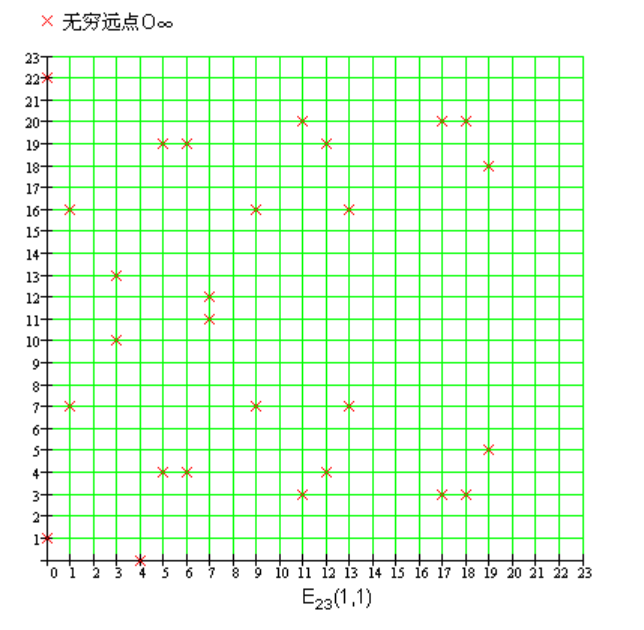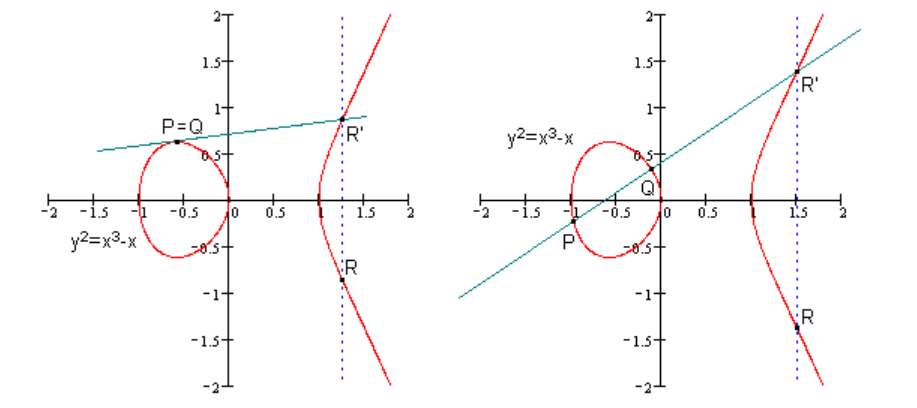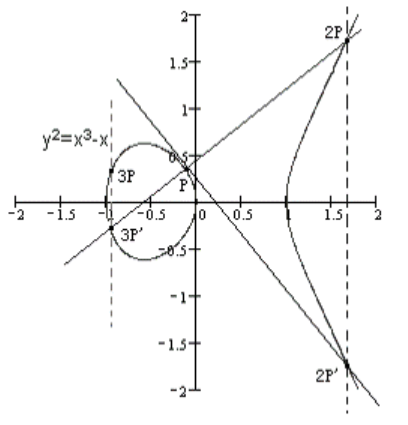1椭圆曲线Ep(a,b)上的点的数目，称为椭圆曲线Ep(a,b)的阶。

2对于椭圆曲线上一点P，如果存在最小的正整数n，使得数乘nP=O∞，则将n称为P的阶，若n不存在，我们说P是无限阶的。事实上，在有限域上定义的椭圆曲线上所有的点的阶n都是存在的，具体证明详见近世代数相关理论。

3对于kG=K，点G是Ep(a,b)上的点，由加法定义，求点K相对容易，并且K也在Ep(a,b)上。但是由K和G，去反推k非常困难，被称为椭圆曲线上离散对数难题。

4描述一个利用椭圆曲线进行加密通信的过程：

(1)用户A选定一条椭圆曲线Ep(a,b)，并取椭圆曲线上一点，作为基点G。
(2)用户A选择一个私有密钥k，并生成公开密钥K=kG。
(3)用户A将Ep(a,b)和点K，G传给用户B。
(4)用户B接到信息后 ，将待传输的明文编码到Ep(a,b)上一点M（编码方法很多，这里不作讨论），并产生一个随机整数r。
(5)用户B计算点C1=M+rK；C2=rG。
(6)用户B将C1、C2传给用户A。
(7)用户A接到信息后，计算C1-kC2，结果就是点M。
因为C1-kC2=M+rK-k(rG)=M+rK-r(kG)=M，再对点M进行解码就可以得到明文。

K=kG（其中 K,G为Ep(a,b)上的点，k为小于n（n是点G的阶）的整数）
不难发现，给定k和G，根据加法法则，计算K很容易；但给定K和G，求k就相对困难了。而且，p越大越困难，但p越大，计算速度会变慢，200位左右可以满足一般安全要求，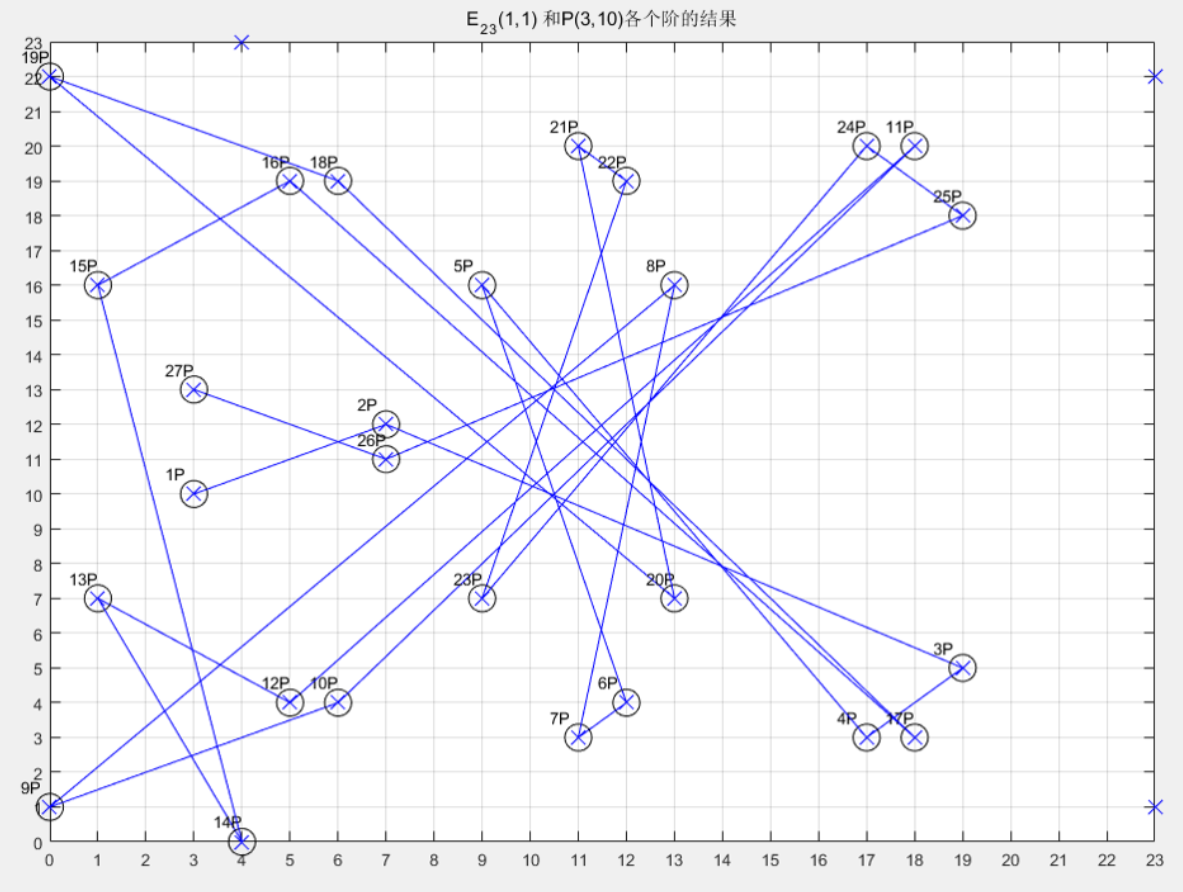p = 0xFFFFFFFF FFFFFFFF FFFFFFFF FFFFFFFF FFFFFFFF FFFFFFFF FFFFFFFE FFFFFC2F
= 2^256 − 2^32 − 2^9 − 2^8 − 2^7 − 2^6 − 2^4 – 1

n = 0xFFFFFFFF FFFFFFFF FFFFFFFF FFFFFFFE BAAEDCE6 AF48A03B BFD25E8C D0364141，全是非常大的数字。

ECC椭圆曲线详解（有具体实例），https://www.cnblogs.com/Kalafinaian/p/7392505.html

ecc算法 swift 相关内容